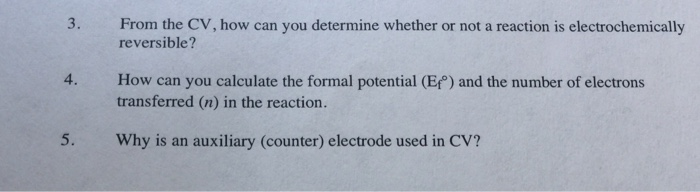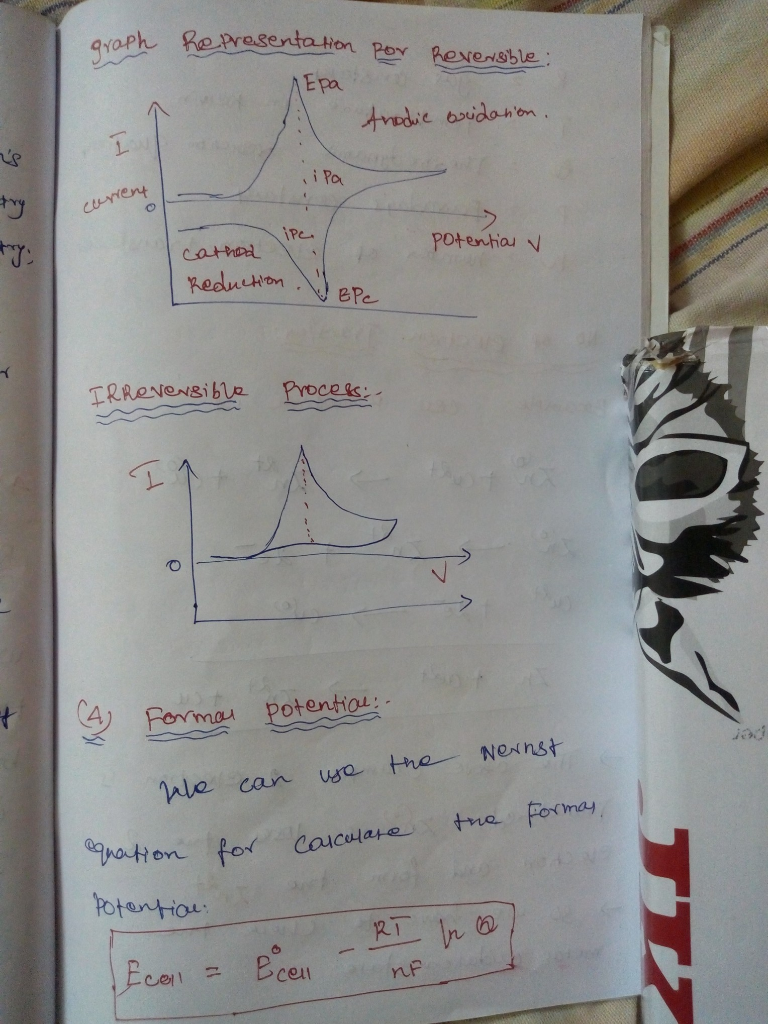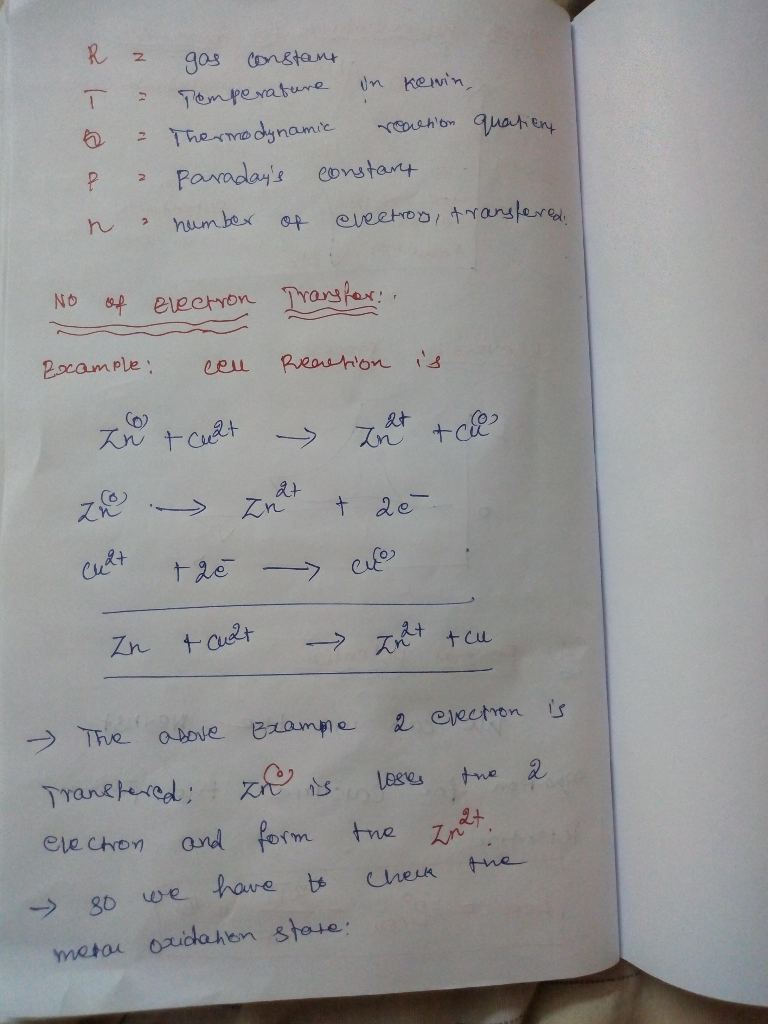# please give full on brief answers for the three following questions 3. From the CV, how can you determine whether or not a reaction is electrochemically 4. How can you calculate the formal potenti...3. From the CV, how can you determine whether or not a reaction is electrochemically 4. How can you calculate the formal potential (Ef) and the number of electrons 5. Why is an auxiliary (counter) electrode used in CV? reversible? transferred (n) in the reaction.

(5) why auxiliary electrode used in CV?

Auxiliary electrode:

The counter electrode ( also known as auxiliary electrode) is an electrode which is used to close the current circuit in the electrochemical cell . It's usually made of an inert material . Eg Pt, Au, graphite, glassy carbon.and usually it doesn't participate in the electrochemical reaction.

Irreversible electron transfer:

If the electron transfer rate is slow, extreme electrode potential are need to drive the electron transfer and register current on the potentiostat. This is known as an irreversible electron transfer.

Reversible electron transfer:

Assuming that both the reduced or oxidised forms of the analyte are infinitely stable in solution, a reversible wave arise when the electron transfer rate is very high.##### Add Answer of: please give full on brief answers for the three following questions 3. From the CV, how can you determine whether or not a reaction is electrochemically 4. How can you calculate the formal potenti...
More Homework Help Questions Additional questions in this topic.